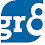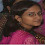# Data Interpretation Example Exercises for SBI PO Exam - Pie Chart

Friends, in our previous posts we have discussed basics and important tips for getting good marks in Data Interpretation of SBI PO Online Exam 2014 along with some examples. You can read that post from here. Now we shall discuss about Pie Charts. Before going into details, lets have a brief look at our Data Interpretation study plan.

Data Interpretation study plan for SBI PO Exam 2014

• Day 1 : Introduction (over)
• Day 2 - 7 : Introductory Practice problems on various types of Data Interpretation models
• Remaining Days upto the exam date : Practice problems of various difficulty levels with shortcuts.
Now let's have a look at an example problem on Pie Chart.

1. In the income section, how many degrees (approx.) should be there in the central angle of the sector representing income tax ?
Solution :
Number of degrees that represent income tax
= ((33/100)x360 ° ) = 118.8 ° = 120 °

2. If an amount of 10 lakh rupees is obtained as excise and custom only, what is the total income of the state ?
Solution :
Total income of the state

= Rs. ((100/4)x10) lakh = Rs. 250 lakh

3. If total expenditure of the state is Rs. 20 crores in a year, how many crores are spent on 'International" ?
Solution :
Amount of money spent on 'International'
= Rs. ((3/100)x20) crore
= Rs. 0.6 Crore

4. What is the ratio of income from sales tax to that from miscellaneous ?
Solution :
Required ratio = 35 : 28 = 5:4

5. What is the ratio of the combined expenditure for defense and agriculture to all other expenditures ?
Solution :
Required ratio = 64 : 36 = 16 : 9

1.Mam,in 1st problem,u,mentioned income tax as 33 why ?in figure it is showing 35madam

1.Typo friend. Corrected now.

2.Solved it Correctly. Thank You.
Ma'am I have one doubt on Syllogisms 'Atleast' Types
Atleast some A are B

1.Mam pls provide Syllogisms 'Atleast' and 'Atmost' type with Explanation

2.atleast=some

atmost=all

3.gr8 work.thnx mam

4.you are god

5.6.i didn't understand 5th problem. can you explain it clearly.

1.The ratio between Defence + Agriculture : All Other Expenses Means(Plan&Non Plan + Interest + International)
i.e. 60+4 : 25+8+3 = 64 : 36 = 16 : 9

2.thank u friend. ....:-)

3.thank u friend

7.8.now i can't use lesson 2 any more ah...?

9.please provide some implicit pi chart question as asked in the last sbi po exam

10.please sir provide the ssc cgl re exam ans sheet link if it is announced

11.Mam More question in pie chart

12.here the questions u provided is very easy level..in sbipo level is very hard so plz provide a good level questions..

13.very nice teaching method....

14.thank so much

15.Thanks for helping...if you would have provided the difficult level with solutions it would have been a great help for us...